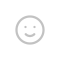## RC滤波电路阻抗为1的电容电感不改变电压与电流的比值，但是改变两者相对相位（偏移了$\frac{\pi}{2}$）

$V_{out}=V_{C}=V_{in}-V_{R}$

$H(jw)=\frac{V_{out}}{V_{in}}=\frac{\frac{1}{jwC}}{R+\frac{1}{jwC}} =\frac{1}{1+jwRC}$

$\left|H(jw)\right|=\frac{1}{\sqrt{1+(\frac{\omega}{\omega_{c}})^{2}}}$

$H(s)=\frac{Y}{X}=\frac{1}{1+s\tau}$

## 数字滤波器

$Y_n=Y_{n-1}+\frac{T_c}{\tau}(X_{n-1}-Y_{n-1})$

$Y_n=aY_{n-1}+(1-a)Y_{n-1}$

double f_c=500; //截止频率
double sampleRate=44100.0; //采样率
double a=f_c/sampleRate; //计算滤波系数
for(int i=1;i<samples.length();i++){
samples[i]=a*samples[i]+(1.0-a)*samples[i-1];//进行一个代的迭
}
//搞定。

https://zhuanlan.zhihu.com/p/479717098

## 发送评论编辑评论Emoji